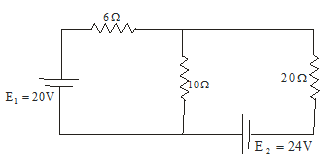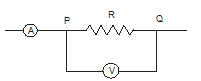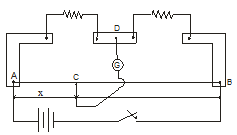# Multiple choice questions on EMF and Electric measurements for JEE Main and Advanced

Question 1
For accurate measurements, the resistance of a voltmeter should be
1. infinite
2. as small as possible
3. as large a possible
4. equal to the resistance across which potential difference is to be measured

Use figure given below for questions 2 to 4
Consider the circuit given belowQuestion 2
The rate at which energy is dissipated is 20Ω resistor
(a) 14.2 Watt
(b) 8.97 Watt
(c) 97.7 Watt
(d) 47.2 Watt
Question 3
Current through 10Ω resistor is
1. 1.54 A
2. 0.67 A
3. 1.22 A
4. 2.21 A
Question 4
Power of battery having emf E1=20V is
1. 44.2 Watt
2. 30.8 Watt
3. 13.4 Watt
4. 20 Watt

Question 5
Consider the figure given belowIf ammeter shows a reading of 10A and voltmeter having internal resistance 3000 Ω measures a voltage of 200V, the resistance R is given by
1. 201.3 Ω
2. 2013 Ω
3. 20.13 Ω
4. 20.00 Ω

Question 6
A constant voltage is applied between the two ends of a uniform metallic wire. Some heat is developed in it. The heat developed is doubled if
(a) both length and radius of wire are halved
(b) both length and radius of wire are doubled
(c) the radius of wire id doubled
(d) length of wire is doubled

Question 7
If a wire of resistance 2Ω is covered with ice and a voltage of 210V is applied across the wire , then rate of melting ice would be
1. 0.85g/s
2. 1.92g/s
3. 6.56g/s
4. none of the above

Question 8
Which of the following statements is/are correct?
1. Both Peltier and Joule effects are reversible
2. Both Peltier and Joule effects are irreversible
3. Joule effect is reversible and Peltier effect is irreversible
4. Joule effect is irreversible and Peltier effect is reversible

Question 9
The arrangement shown below is of the meter bridge experiment. Here AC=x corresponds to the null deflection in the galvanometer. What will be the value of AC if the radius of the wire AB is doubled?1. x/2
2. x/4
3. 2x
4. x

Assertion Reason type questions
1. Both assertion and reason are true and reason is correct explanation of assertion
2. Both assertion and reason are true and reason is not the correct explanation of assertion
3. Assertion is true but reason is false
4. Both assertion and reason are false.

Question 10
Assertion
The distribution of currents within the network for a given amount of current always takes place in a way that leads to minimum total power distribution in the circuit
Reason
For maximum output current by a source the source resistance must be equal to external load resistance.

Question 11
Assertion
When temperature of a cold junction of a thermocouple is lowered , the value of neutral temperature of this thermocouple rises
Reason
More thermo emf is produced when temperature difference between two junctions of thermocouple is raised.

Question 12
Assertion
A domestic appliance, working on a three pin , will continue working even if the top pin is removed
Reason
Third pin is used as a safety device.

Question 13
Assertion
A potentiometer of larger length is used for accurate measurements
Reason
Potential gradient for potentiometer of larger length with a given battery becomes small.